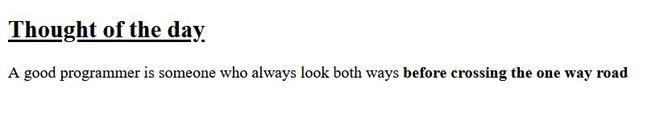Skip to content
Related Articles
How to bold the text using CSS ?
• Difficulty Level : Easy
• Last Updated : 15 Mar, 2021

We know that in HTML, we have <b> and <strong> tags to make the content bold. When it comes to making a piece of text bold using CSS, then also we have an appropriate property to do the same.

We will use the font-weight property of CSS to make the content bold. We have a variety of options to set the thickness level of our text.

• normal : It is the normal font-weight.  It is the same as 400, the default numeric-value for boldness.
• bold : It is the bold font-weight. It is the same as 700.
• bolder : It sets the font-weight bolder than the parent element.
• lighter : It sets the font-weight lighter than the parent element
• <number>: A <number> value between 1 and 1000, inclusive (in increasing order of boldness level).

When lighter or bolder is specified, the below chart shows how the absolute font-weight of the element is determined.

Example 1: The following example demonstrates a simple text which is represented in bold using CSS font-weight property.

## HTML

 ```<``html``>``    ``<``head``>``        ``<``style` `type``=``"text/css"``>``            ``h2 {``                ``font-weight: 700;``                ``color: green;``            ``}``            ``.text {``                ``font-weight: bold;``            ``}``        ````    ````    ``<``body``>``        ``<``h2``>``          ``Welcome To Geeks for Geeks``        ````        ``<``p` `class``=``"text"``>``          ``A Computer Science portal for geeks``        ````    `````

Output:Example 2: The following example demonstrates few simple texts represented using other font-weight properties.

## HTML

 ```<``html``>``    ``<``body``>``        ``<``h2` `style="font-weight: bold;``                   ``text-decoration: underline;">``            ``Thought of the day``        ````        ``<``p` `style``=``"font-weight: lighter;"``>``          ``A good programmer is someone who ``          ``always look both ways``          ``<``span` `style``=``"font-weight: 900;"``>``            ``before crossing the one way road. ``          ````        ````    `````

Output:font-weight property

My Personal Notes arrow_drop_up